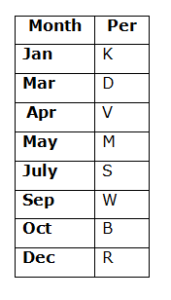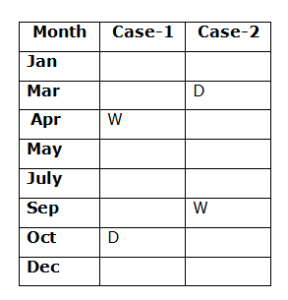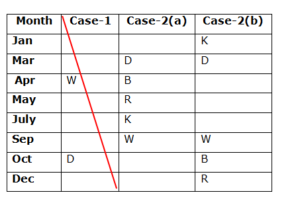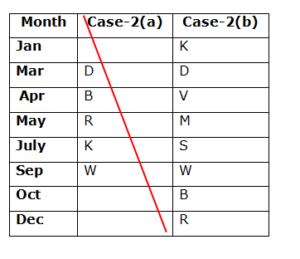# CWC/FCI Prelims 2019 Reasoning Ability Questions (Day-43)

Dear Readers, Exam Race for the Year 2019 has already started, To enrich your preparation here we are providing new series of Practice Questions on CWC/FCI Prelims 2019 Reasoning Ability Questions on daily basis. Aspirants, practice these CWC/FCI Prelims 2019 Reasoning Ability Questions (Day-43) on a regular basis to improve your score in the aptitude section. Start your effective preparation from the right beginning to get success in the upcoming CWC/FCI Exam.

[WpProQuiz 6065]

Direction (1-5): Read the following information carefully and answer the questions given below.

Eight persons B, D, K, M, R, S, V and W are born in different months viz., January, March, April, May, July, September, October and December of the same year but not necessary in the same order.

Three persons are born between D and W. W does not born in the month which has odd number of days. R was born immediately after B. As many persons born before R is same as after K. As many persons born between B and S is same as the persons born between D and M. M is not younger than both S and W.

1) Four of the following five are alike in a certain way and hence form a group. Which one of the following that does not belong to the group?

a) K

b) R

c) S

d) V

e) M

2) V was born in which of the following months?

a) September

b) October

c) December

d) April

e) May

3) How many persons are born between M and R?

a) One

b) Two

c) Three

d) None

e) More than three

4) Who among the following is the youngest person?

a) R

b) K

c) D

d) S

e) W

5) Which of the following statements is true?

a) B was born in April

b) Only one person was born before D

c) No one was born between S and V

d) More than three persons born between W and V

e) None is true

Direction (6-10): In these questions, a relationship between different elements is shown in the statements. The statements are followed by two conclusions.

a) If only conclusion I follows.

b) If only conclusion II follows

c) If either conclusion I or II follows

d) If neither conclusion I nor II follows

e) If both conclusion I and II follows

6) Statements: P≥D>S=H≥N; Q≤D=M<K.

Conclusions:

I. M>N

II. P>K

7) Statements: H<J≤M=T≥P; K≥L=T<Q≤X.

Conclusions:

I. H<Q

II. P≤K

8) Statements: L=P≥F<Z≤B; M≥Y>B≤A=T.

Conclusions:

I. F≤A

II. T>P

9) Statements: J≤S<H=U≥N; B=V≥L≥S>Y.

Conclusions:

I. B>U

II. Y<U

10) Statements: P≥O=Y≤T<R; V≤B≥T>N=M.

Conclusions:

I. O≤V

II. M<Y

Direction (1-5):• Three persons are born between D and W. W does not born in the month which has odd number of days.• R was born immediately after B. As many persons born before R is same as after K.
• So, Case-1 will be dropped.• As many persons born between B and S is same as the persons born between D and M. M is not youngerthan both S and W.

So, Case-2(a) will be dropped.1) Answer: d)

Directions (6-10):

I. M>N → M=D>S=H≥N

II. P>K → P≥D=M<K

I. H<Q → H<J≤M=T<Q

II. P≤K → P≤T=L≤K

I. F≤A → F<Z≤B≤A

II. T>P → T=A≥B≥Z>F≤P

I. B>U → B=V≥L≥S<H=U

II. Y<U → Y<S<H=U